# A Brief History of polyval

When I first started working at MathWorks, there were already a few functions in MATLAB for working with polynomials. One of these, polyval, for polynomial evaluation, used one algorithm, based on Horner's rule, known for its efficiency, especially suitable for embedded controller environments. After a customer wondered why the function took so long to evaluate a given polynomial for a scalar input value x, we updated polyval to use a faster algorithm for the scalar case, and that algorithm uses the function filter. I thought I'd discuss a few more details here.

Note: since I wrote this post (but before I published it) there was a discussion on the newsgroup about this same topic. Be sure to read the reply from John D'Errico.

### Horner's Rule

Let's first look at the code for evaluating a polynomial for multiple values of input x. Here's the relevant portion of polyval, nc is the length of the vector representing the polynomial (one more than the polynomial degree).

dbtype polyval 62:67
62    % Use Horner's method for general case where X is an array.
63    y = zeros(siz_x, superiorfloat(x,p));
64    if nc>0, y(:) = p(1); end
65    for i=2:nc
66        y = x .* y + p(i);
67    end



Here's what a general polynomial looks like: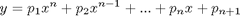This equation can be rewritten like this,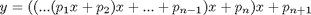and is the basis for evaluating the polynomial for an array x. Notice that the formula basically starts with the coefficient of the highest degree (named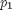) multiplied by x, then adds the next coefficient, multiplies that result by x again, adds the next coefficient, and so on, until reaching the final coefficient (the constant term, for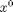). In this formulation, we never need to explicitly create all the powers of x to evaluate the polynomial.

### The Scalar Case, Using filter

Here's the code for the scalar evalation case using the function filter.

dbtype polyval 52:53
52        y = filter(1,[1 -x],p);
53        y = y(nc);



It may not, at first glance, appear relevant to polynomial evaluation. However, taking a close look at the mathematics reveals that it indeed can be useful. First, let's examine the filter formula. In this version, the coefficients for a have been normalized so a(1) = 1 (which is true for the inputs to filter you've just seen).

Now, let's try a small example. Suppose we want to evaluate the same third degree polynomial I used in another set of posts recently.

p = [1 0 1 -1];

which represents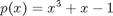When you call filter like this y = filter(b,a,x), the results are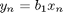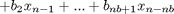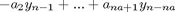Looking again at the polyval code, use these substitutions for the inputs to filter: b = 1, a = [1 -x], and x = p. We evaluate the filter for the values p, the polynomial coefficients! Let's do a little math to see why filter works. Following the formula for the output of the function filter, and assuming p(0) = 0, we can find the first output.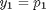And here are the rest, going up to the final coefficient in our example.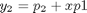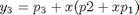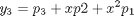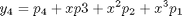It sure looks like the final answer here is the polynomial p evaluated at x.

### How Close are the Algorithms?

Numerically, the two algorithms end up being essentially the same.

x = 0:0.001:1;
resultArray = polyval(p,x);
resultScalar = zeros(size(x));
for index = 1:numel(x)
resultScalar(index) = polyval(p,x(index));
end
allequal = isequal(resultArray,resultScalar)
allequal =
1


Identical!

### Timing

I mentioned we made this change for speed. This particular code is tricky to check timing since, especially if the polynomial degree is small, the number of mathematic operations can be swamped by the timing mechanism. When computers were slower, and before we increased performance of for-loops in MATLAB, measuring the performance differences between these code alternatives was much easier than it is today.

### Multiple Algorithms

Do you try to write your code as one size fits all, or do you create code in which you switch algorithms or implementations, such as we've seen in polyval, to account for different conditions such as array size? I'd love to hear more about your experiences with these ideas here.

Published with MATLAB® 7.8

|